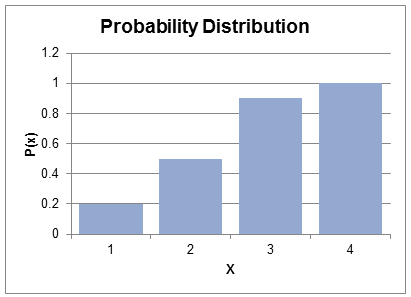# Discrete Vs. Continuous Random Variable

### Discrete Random Variable

A random variable is said to be discrete if the total number of values it can take can be counted. Alternatively, we can say that a discrete random variable can take only a discrete countable value such as 1, 2, 3, 4, etc. For example, in case of the roll of a die, there could be only 6 outcomes. This is an example of a discrete random variable. Similarly, if we have to pick a stock from S&P 500 index, it’s a discrete random variable. Each outcome should also have a positive probability.

Let’s say a variable X can take values 1, 2, 3, 4. The probabilities of each of these outcomes are given below:

 xi P(xi) 1 0.2 2 0.3 3 0.4 4 0.1

We can draw this distribution in the form of a histogram.Note: What would be the probability of the random variable X being equal to 5?

P(5) = 0 because as per our definition the random variable X can only take values, 1, 2, 3 and 4.

### Continuous Random Variable

In contrast to discrete random variable, a random variable will be called continuous if it can take an infinite number of values between the possible values for the random variable. Examples include measuring the height of a person, or the amount of rain fall that a city receives. The number of possible outcomes is infinite. In that case, what is the probability that the random variable X will get a certain value x?

P(x) will be 0 because we are talking about the possibility of one outcome from an infinite number of outcomes.

In finance, some variables such as price change of a stock, or the returns earned by an investor are considered to be continuous, even though they are actually discrete, because the number of possible outcomes is large, and the probability of each outcome is very small. For example, the probability of an investor earning a return of exactly 8.25% is almost zero.

The probability distribution of a continuous random variable is called probability density function.

Get our Data Science for Finance Bundle for just $29$51.